login  home  contents  what's new  discussion  bug reports help  links  subscribe  changes  refresh  edit

## Computing with non-commutative polynomials

### Univariate differential case

Let's first consider a differential operator algebra.

fricas
Z ==> Integer
Type: Void
fricas
P ==> UnivariatePolynomial('x, Z)
Type: Void
fricas
sigma1: Automorphism P := 1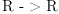(1)
Type: Automorphism(UnivariatePolynomial(x,Integer))
fricas
delta1: P -> P := D$P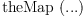(2) Type: (UnivariatePolynomial(x,Integer) -> UnivariatePolynomial(x,Integer)) fricas S1 ==> UnivariateSkewPolynomial('X, P, sigma1, delta1) Type: Void fricas x1: S1 := 'x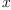(3) Type: UnivariateSkewPolynomial?(X,UnivariatePolynomial(x,Integer),R -> R,theMap(DIFRING-;D;2S;1,303)) fricas X1: S1 := 'X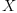(4) Type: UnivariateSkewPolynomial?(X,UnivariatePolynomial(x,Integer),R -> R,theMap(DIFRING-;D;2S;1,303)) fricas X1*x1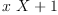(5) Type: UnivariateSkewPolynomial?(X,UnivariatePolynomial(x,Integer),R -> R,theMap(DIFRING-;D;2S;1,303)) ## Univariate shift case Similarly, we can define a shift algebra. fricas xp: P := 'x(6) Type: UnivariatePolynomial(x,Integer) fricas sigma2: Automorphism P := morphism((p: P): P +-> p(x+1), (p: P): P +-> p(x-1))(7) Type: Automorphism(UnivariatePolynomial(x,Integer)) fricas delta2: P -> P := (p: P): P +-> 0(8) Type: (UnivariatePolynomial(x,Integer) -> UnivariatePolynomial(x,Integer)) fricas S2 ==> UnivariateSkewPolynomial('X, P, sigma2, delta2) Type: Void fricas x2: S2 := 'x(9) Type: UnivariateSkewPolynomial?(X,UnivariatePolynomial(x,Integer),R -> R,theMap(*1;anonymousFunction;2;initial;internal)) fricas X2: S2 := 'X(10) Type: UnivariateSkewPolynomial?(X,UnivariatePolynomial(x,Integer),R -> R,theMap(*1;anonymousFunction;2;initial;internal)) fricas X2*x2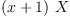(11) Type: UnivariateSkewPolynomial?(X,UnivariatePolynomial(x,Integer),R -> R,theMap(*1;anonymousFunction;2;initial;internal)) ## Multivariate case The multivariate case is only sligthly more complicated. Let us here use a field as coefficient domain. fricas Q ==> Fraction UnivariatePolynomial('q, Z) Type: Void fricas VM ==> OrderedVariableList ['y, 'w, 'u] Type: Void fricas V ==> OrderedVariableList ['Y, 'W, 'U] Type: Void fricas M ==> SparseMultivariatePolynomial(Q, VM) Type: Void fricas K := Fraction M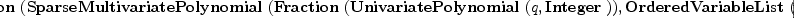(12) Type: Type fricas sigmay: Automorphism K := 1(13) Type: Automorphism(Fraction(SparseMultivariatePolynomial?(Fraction(UnivariatePolynomial(q,Integer)),OrderedVariableList([y,w,u])))) fricas yv: VM := 'y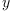(14) Type: OrderedVariableList([y,w,u]) fricas derivy(m: M): M == D(m, yv) Function declaration derivy : SparseMultivariatePolynomial(Fraction( UnivariatePolynomial(q,Integer)),OrderedVariableList([y,w,u])) -> SparseMultivariatePolynomial(Fraction(UnivariatePolynomial(q, Integer)),OrderedVariableList([y,w,u])) has been added to workspace. Type: Void fricas deltay(k: K): K == D(k, derivy) Function declaration deltay : Fraction(SparseMultivariatePolynomial( Fraction(UnivariatePolynomial(q,Integer)),OrderedVariableList([y, w,u]))) -> Fraction(SparseMultivariatePolynomial(Fraction( UnivariatePolynomial(q,Integer)),OrderedVariableList([y,w,u]))) has been added to workspace. Type: Void fricas wm: M := 'w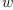(15) Type: SparseMultivariatePolynomial?(Fraction(UnivariatePolynomial(q,Integer)),OrderedVariableList([y,w,u])) fricas sigmaw1(k: K): K == eval(k, wm, wm+1) Function declaration sigmaw1 : Fraction(SparseMultivariatePolynomial (Fraction(UnivariatePolynomial(q,Integer)),OrderedVariableList([y ,w,u]))) -> Fraction(SparseMultivariatePolynomial(Fraction( UnivariatePolynomial(q,Integer)),OrderedVariableList([y,w,u]))) has been added to workspace. Type: Void fricas sigmaw2(k: K): K == eval(k, wm, wm-1) Function declaration sigmaw2 : Fraction(SparseMultivariatePolynomial (Fraction(UnivariatePolynomial(q,Integer)),OrderedVariableList([y ,w,u]))) -> Fraction(SparseMultivariatePolynomial(Fraction( UnivariatePolynomial(q,Integer)),OrderedVariableList([y,w,u]))) has been added to workspace. Type: Void fricas sigmaw: Automorphism K := morphism(sigmaw1, sigmaw2) fricas Compiling function sigmaw1 with type Fraction( SparseMultivariatePolynomial(Fraction(UnivariatePolynomial(q, Integer)),OrderedVariableList([y,w,u]))) -> Fraction( SparseMultivariatePolynomial(Fraction(UnivariatePolynomial(q, Integer)),OrderedVariableList([y,w,u]))) fricas Compiling function sigmaw2 with type Fraction( SparseMultivariatePolynomial(Fraction(UnivariatePolynomial(q, Integer)),OrderedVariableList([y,w,u]))) -> Fraction( SparseMultivariatePolynomial(Fraction(UnivariatePolynomial(q, Integer)),OrderedVariableList([y,w,u])))(16) Type: Automorphism(Fraction(SparseMultivariatePolynomial?(Fraction(UnivariatePolynomial(q,Integer)),OrderedVariableList([y,w,u])))) fricas um: M := 'u; qQ: Q := 'q::Q; qQ1 := inv qQ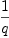(17) Type: Fraction(UnivariatePolynomial(q,Integer)) fricas sigmau1(k: K): K == eval(k, um, qQ*um)$K
Function declaration sigmau1 : Fraction(SparseMultivariatePolynomial
(Fraction(UnivariatePolynomial(q,Integer)),OrderedVariableList([y
,w,u]))) -> Fraction(SparseMultivariatePolynomial(Fraction(
UnivariatePolynomial(q,Integer)),OrderedVariableList([y,w,u])))
has been added to workspace.
Type: Void
fricas
sigmau2(k: K): K == eval(k, um, qQ1*um)$K Function declaration sigmau2 : Fraction(SparseMultivariatePolynomial (Fraction(UnivariatePolynomial(q,Integer)),OrderedVariableList([y ,w,u]))) -> Fraction(SparseMultivariatePolynomial(Fraction( UnivariatePolynomial(q,Integer)),OrderedVariableList([y,w,u]))) has been added to workspace. Type: Void fricas sigmau: Automorphism K := morphism(sigmau1, sigmau2)$Automorphism(K)
fricas
Compiling function sigmau1 with type Fraction(
SparseMultivariatePolynomial(Fraction(UnivariatePolynomial(q,
Integer)),OrderedVariableList([y,w,u]))) -> Fraction(
SparseMultivariatePolynomial(Fraction(UnivariatePolynomial(q,
Integer)),OrderedVariableList([y,w,u])))
fricas
Compiling function sigmau2 with type Fraction(
SparseMultivariatePolynomial(Fraction(UnivariatePolynomial(q,
Integer)),OrderedVariableList([y,w,u]))) -> Fraction(
SparseMultivariatePolynomial(Fraction(UnivariatePolynomial(q,
Integer)),OrderedVariableList([y,w,u])))(18)
Type: Automorphism(Fraction(SparseMultivariatePolynomial?(Fraction(UnivariatePolynomial(q,Integer)),OrderedVariableList([y,w,u]))))
fricas
deltawu(k: K): K == 0
Function declaration deltawu : Fraction(SparseMultivariatePolynomial
(Fraction(UnivariatePolynomial(q,Integer)),OrderedVariableList([y
,w,u]))) -> Fraction(SparseMultivariatePolynomial(Fraction(
UnivariatePolynomial(q,Integer)),OrderedVariableList([y,w,u])))
has been added to workspace.
Type: Void
fricas
YV: V := index(1)$V; WV: V := index(2)$V;
Type: OrderedVariableList([Y,W,U])
fricas
sigma(v: V): Automorphism K ==_
if v=YV then sigmay else if v=WV then sigmaw else sigmau
Function declaration sigma : OrderedVariableList([Y,W,U]) ->
Automorphism(Fraction(SparseMultivariatePolynomial(Fraction(
UnivariatePolynomial(q,Integer)),OrderedVariableList([y,w,u]))))
has been added to workspace.
Type: Void
fricas
delta(v: V): K -> K == if v=YV then deltay else deltawu
Function declaration delta : OrderedVariableList([Y,W,U]) -> (
Fraction(SparseMultivariatePolynomial(Fraction(
UnivariatePolynomial(q,Integer)),OrderedVariableList([y,w,u])))
-> Fraction(SparseMultivariatePolynomial(Fraction(
UnivariatePolynomial(q,Integer)),OrderedVariableList([y,w,u]))))
has been added to workspace.
Type: Void
fricas
T ==> SparseMultivariateSkewPolynomial(K, V, sigma, delta)
Type: Void
fricas
y: T := 'y::K::T; w: T := 'w::K::T; u: T := 'u::K::T; q: T := qQ::K::T;
fricas
Compiling function sigma with type OrderedVariableList([Y,W,U]) ->
Automorphism(Fraction(SparseMultivariatePolynomial(Fraction(
UnivariatePolynomial(q,Integer)),OrderedVariableList([y,w,u]))))
fricas
Compiling function delta with type OrderedVariableList([Y,W,U]) -> (
Fraction(SparseMultivariatePolynomial(Fraction(
UnivariatePolynomial(q,Integer)),OrderedVariableList([y,w,u])))
-> Fraction(SparseMultivariatePolynomial(Fraction(
UnivariatePolynomial(q,Integer)),OrderedVariableList([y,w,u]))))
Type: SparseMultivariateSkewPolynomial?(Fraction(SparseMultivariatePolynomial?(Fraction(UnivariatePolynomial(q,Integer)),OrderedVariableList([y,w,u]))),OrderedVariableList([Y,W,U]),theMap(*1;sigma;1;initial),theMap(*1;delta;1;initial))
fricas
Y: T := monomial(1, index(1)$V, 1)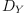(19) Type: SparseMultivariateSkewPolynomial?(Fraction(SparseMultivariatePolynomial?(Fraction(UnivariatePolynomial(q,Integer)),OrderedVariableList([y,w,u]))),OrderedVariableList([Y,W,U]),theMap(*1;sigma;1;initial),theMap(*1;delta;1;initial)) fricas W: T := monomial(1, index(2)$V, 1)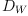(20)
Type: SparseMultivariateSkewPolynomial?(Fraction(SparseMultivariatePolynomial?(Fraction(UnivariatePolynomial(q,Integer)),OrderedVariableList([y,w,u]))),OrderedVariableList([Y,W,U]),theMap(*1;sigma;1;initial),theMap(*1;delta;1;initial))
fricas
U: T := monomial(1, index(3)\$V, 1)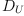(21)
Type: SparseMultivariateSkewPolynomial?(Fraction(SparseMultivariatePolynomial?(Fraction(UnivariatePolynomial(q,Integer)),OrderedVariableList([y,w,u]))),OrderedVariableList([Y,W,U]),theMap(*1;sigma;1;initial),theMap(*1;delta;1;initial))
fricas
t: T := y*w*u*q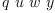(22)
Type: SparseMultivariateSkewPolynomial?(Fraction(SparseMultivariatePolynomial?(Fraction(UnivariatePolynomial(q,Integer)),OrderedVariableList([y,w,u]))),OrderedVariableList([Y,W,U]),theMap(*1;sigma;1;initial),theMap(*1;delta;1;initial))
fricas
Y*t
fricas
Compiling function deltay with type Fraction(
SparseMultivariatePolynomial(Fraction(UnivariatePolynomial(q,
Integer)),OrderedVariableList([y,w,u]))) -> Fraction(
SparseMultivariatePolynomial(Fraction(UnivariatePolynomial(q,
Integer)),OrderedVariableList([y,w,u])))
fricas
Compiling function derivy with type SparseMultivariatePolynomial(
Fraction(UnivariatePolynomial(q,Integer)),OrderedVariableList([y,
w,u])) -> SparseMultivariatePolynomial(Fraction(
UnivariatePolynomial(q,Integer)),OrderedVariableList([y,w,u]))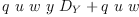(23)
Type: SparseMultivariateSkewPolynomial?(Fraction(SparseMultivariatePolynomial?(Fraction(UnivariatePolynomial(q,Integer)),OrderedVariableList([y,w,u]))),OrderedVariableList([Y,W,U]),theMap(*1;sigma;1;initial),theMap(*1;delta;1;initial))
fricas
W*t
fricas
Compiling function deltawu with type Fraction(
SparseMultivariatePolynomial(Fraction(UnivariatePolynomial(q,
Integer)),OrderedVariableList([y,w,u]))) -> Fraction(
SparseMultivariatePolynomial(Fraction(UnivariatePolynomial(q,
Integer)),OrderedVariableList([y,w,u])))(24)
Type: SparseMultivariateSkewPolynomial?(Fraction(SparseMultivariatePolynomial?(Fraction(UnivariatePolynomial(q,Integer)),OrderedVariableList([y,w,u]))),OrderedVariableList([Y,W,U]),theMap(*1;sigma;1;initial),theMap(*1;delta;1;initial))
fricas
U*t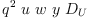(25)
Type: SparseMultivariateSkewPolynomial?(Fraction(SparseMultivariatePolynomial?(Fraction(UnivariatePolynomial(q,Integer)),OrderedVariableList([y,w,u]))),OrderedVariableList([Y,W,U]),theMap(*1;sigma;1;initial),theMap(*1;delta;1;initial))

fricas
)set message time on
(Y+y+1)^100;
Type: SparseMultivariateSkewPolynomial?(Fraction(SparseMultivariatePolynomial?(Fraction(UnivariatePolynomial(q,Integer)),OrderedVariableList([y,w,u]))),OrderedVariableList([Y,W,U]),theMap(*1;sigma;1;initial),theMap(*1;delta;1;initial))
fricas
Time: 0.50 (EV) = 0.50 sec
(X1+x1+1)^100;
Type: UnivariateSkewPolynomial?(X,UnivariatePolynomial(x,Integer),R -> R,theMap(DIFRING-;D;2S;1,303))
fricas
Time: 0.25 (EV) = 0.25 sec
(X2+x2+1)^100;
Type: UnivariateSkewPolynomial?(X,UnivariatePolynomial(x,Integer),R -> R,theMap(*1;anonymousFunction;2;initial;internal))
fricas
Time: 0.82 (IN) + 0.70 (EV) + 0.11 (OT) = 1.63 sec

 Subject:   Be Bold !! ( 14 subscribers )